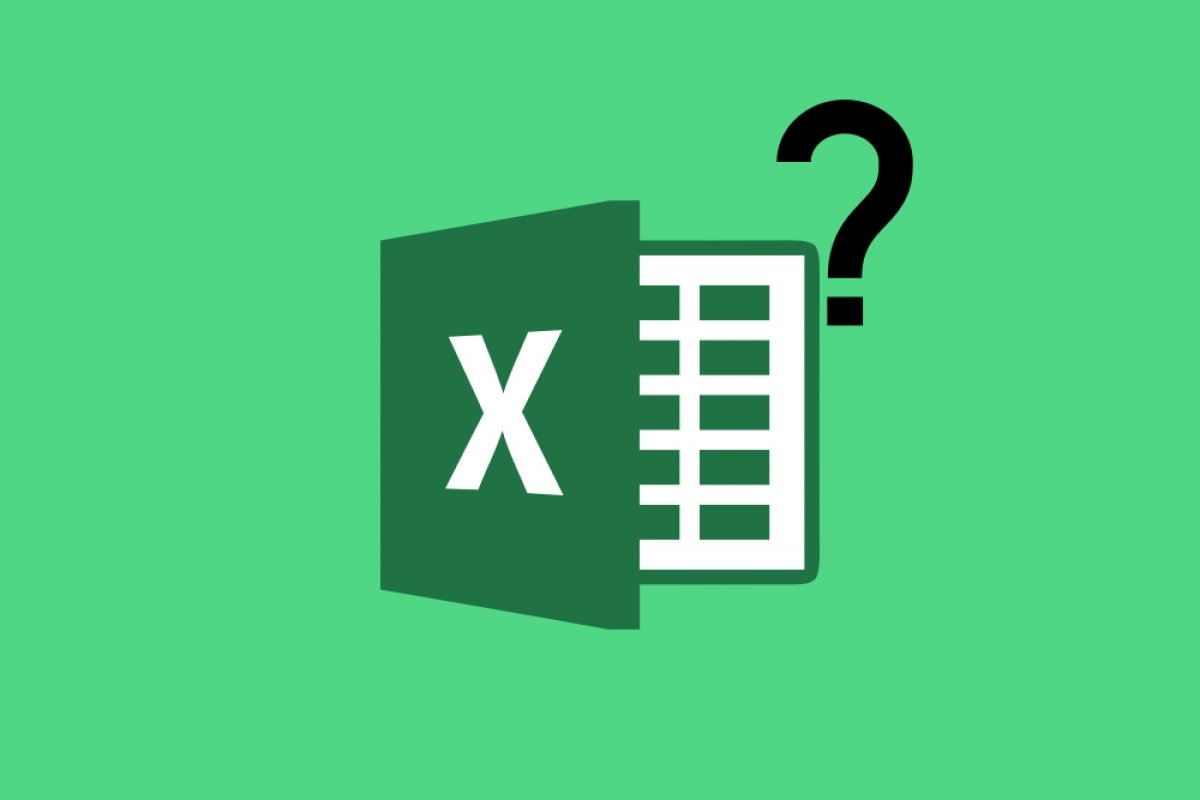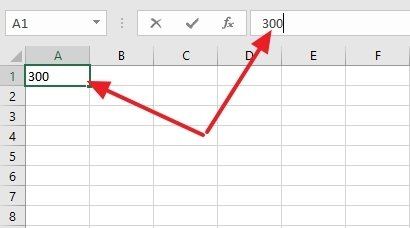A spreadsheet is an application that allows us to handle numeric and alphanumeric data, which are arranged in tables composed of cells. Each cell is a unit of information where values and formulas can be inserted to perform mathematical calculations. Microsoft Excel is the program designed to work with them so we can keep information and records more easily.

These spreadsheets are part of what is known as a workbook, and there may be one or more in each workbook. At the same time, each sheet is made up of columns (distinguished by the letters of the alphabet) and rows (identified by numbers). This system allows you to locate a specific cell by referring to it by the letter of the column and the number of the row where it is located (for example, H6).

Although we may think that we are entering data in each cell independently by double-clicking on it, in reality, it is done in a matrix that can be found just below the main toolbar:Entering data in the column and the matrix

In this matrix, we can write the numeric or alphanumeric data we want to store in that cell, even a function if necessary. The matrix is not limited in the extent of its contents.

In a spreadsheet such as Excel, we can also perform actions on a group of rows and columns through basic selection, copy, cut, paste, and delete actions.

The contents of these spreadsheets can be exported to relational database systems, where each column would be the equivalent of a table. Excel also allows the information to be represented visually through the use of graphs.